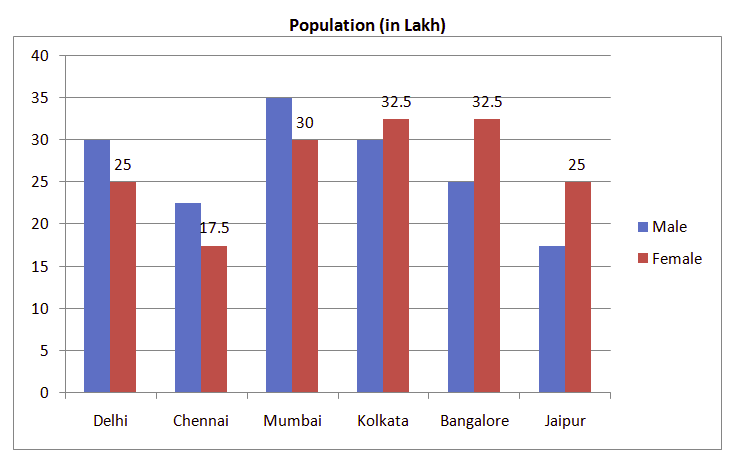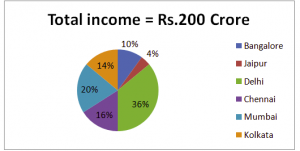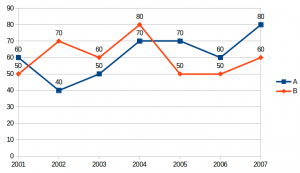## Data Interpretation For SBI PO : Set – 51

D.1-5) Working male and female population (in lakh) in various cities1) What is the difference between the number of working females in Bangalore and the number of working males in Chennai?

a) 12.5 lakh

b) 11 lakh

c) 9 lakh

d) 12 lakh

e) 10 lakh

e)

The difference between the working females in Bangalore and the working males in Chennai = 32.5 ⎯ 22.5 = 10 lakh

2) In which city is the income per working person the minimum?

a) Delhi

b) Jaipur

d) Chennai

e) Mumbai

b)

Income per working person = Total income of city / Number of working people in city

Income per working person in Delhi = 200 Crore x 36/100  /  (30+25) Lakh = 72 / 55 = Rs.130.9

In Chennai = 200 x 16/100  /  (22.5+17.5) Lakh = Rs.80

In Mumbai = 200 x 20/100  /  (35+30) Lakh = Rs.61.53

In Kolkata = 200 x 14/100  /  (30+32.5) Lakh = Rs.44.8

In Bangalore = 200 x 10/100  /  (25+32.5) Lakh = Rs.34.78

In Jaipur = 200 x 4/100  /  (17.5+25) Lakh = Rs.18.82

The income per working person in Jaipur is the minimum.

3) What is the sum of the average working male and average working female population of the given six cities (calculate approximate value)?

a) 63.35 lakh

b) 49.96 lakh

c) 51.48 lakh

d) 53.75 lakh

e) 65.51 lakh

d)

Average number of working males = 1/6 × (30 + 22.5 + 35 + 30 + 25 + 17.5) = 26.66 lakh

Average number of working females = 1/6 × (25 + 17.5 + 30 + 32.5 + 32.5 + 25) =27.08 lakh

So, required sum = 26.66 + 27.08 = 53.75 lakh

4) In Delhi, what is the difference between the income of males and that of females? (Assume each person (male/female) has equal income.)

a) Rs.6.545 Crore

b) Rs.5.055 Crore

c) Rs.2.935 Crore

d) Rs.3.455 Crore

e) Rs.4.565 Crore

a)

Total income of Delhi = [200 ×36/100] = Rs.72 Crore

Income per person = 72 Crore / 55 Lakh = Rs.130.9

So, required difference of income = 5 lakh × 130.9 = Rs.654.5 lakh

= Rs.6.545 Crore

5) The number of working females in Mumbai is what percent of the number of working males in Bangalore?

a) 95%

b) 110%

c) 120%

d) 132%

e) 144%

c)

Required % = 30/25 × 100 = 120%

D.6-10) Investments in Lakh of two business partners A and B over the year6. What was the per cent rise in A’s investment in 2004 from the previous year ?

a) 25%

b) 20%

c) 331.3%

d) 332.3%

e) None of these

e)

% rise = (70 – 50/50)*100 = 60%

7. What was the per cent rise in investment of B in 2004 from 2001 ?

a) 45·6

b) 37·5

c) 30

d) 60

e) None of these

d)

% rise = (70-50/50)*100 = 60%

8. What was the per cent rise/fall in the total investment of A and B together from 2002 to 2005 ? (Rounded off to two digits after decimal)

a) 8·33% fall

b) 9·09% rise

c) 8·33% rise

d) 9·09% fall

e) None of these

b)

% rise = (50+70) -(40+70)/(40+70)*100 = 10*100/110=9.09%

9. What is the ratio between total investment of A in 2001, 2002 and 2003 together and the total investment of B in these three years together respectively ?

a) 5 : 6

b) 6 : 5

c) 15 : 17

d) 17 : 15

e) None of these

a)

Required Ratio = (60+40+50)/(50+70+60) = 150/180 = 5:6

10. Investment of B in 2003 is approximately what per cent of his total investment for all the years together ?

a) 12

b) 18

c) 20

d) 17

e) 14